Add marks (+, -, *, /, brackets) to fullfill equations

1 3 6 5 = 10

This is for the 4th grade of the primary school - with no negative numbers yet

x =  10

### Step-by-step explanation: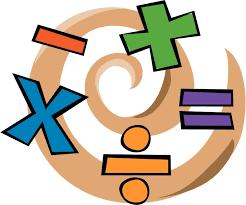Did you find an error or inaccuracy? Feel free to write us. Thank you!Tips to related online calculators
Do you have a linear equation or system of equations and looking for its solution? Or do you have a quadratic equation?

## Related math problems and questions:

• Pupil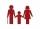I'm a primary school pupil. I attended the parents' exercises with children 1/4 of my age, 1/3 for drawing, and 1/6 for flute. For the first three years of my life, I had no ring, and I never went to two rings simultaneously. How old am I?Why does 1 3/4 + 2 9/10 equal 4.65? How do you solve this?Add two mixed fractions: 2 4/6 + 1 3/6
• Evaluate 11Multiply the quotient of 6 and 2 by 3, then add 1. Add 1 and 6, then divide by 2 and multiply by 3. Divide 6 by the product of 2 and 3, then add 1.
• Expression 1What is 7+8-(5×2)+5-4+(6×(5-3)+6)-(8+10)-7+6?
• Expression 8Evaluate this expressions: a) 5[3 + 4(2.8 - 3)] b) 5×(8-4)÷4-2
• Brick weightThe brick weighs 3 kg and a half-brick. How much does one brick weigh?
• Identity123456789 = 100 Use only three plus or minus characters to correct previous identity/equation.
• Cake fractions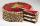Thomas ate 1/3 of cake, Bohouš of the rest of the cake ate 2/5. What fraction of cake left over for others?
• GroupA group of kids wanted to ride. When the children were divided into groups of 3 children, one remain. When divided into groups of 4 children, 1 remains. When divided into groups of 6 children, 1 remains. After divided into groups of 5 children, no one lef
• Sum three fractionsWork out the sum of 1/4, 1/5 and 3/10.
• With bracket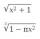Calculate (evaluate) simple mathematical expression with a negative numbers and a bracket: 13+15*5-2*(-6)Add this two mixed numbers: 1 5/6 + 2 2/11=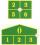Fill in the examples correctly brackets: A) 7.9+12:3-2 =23 B) 7.9+12:3-2=75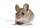3 cats eat 3 mice in 3 days. How many mice will be eaten by 10 cats in 10 days?Place one pair of brackets in expression 20-16 + 10 - 4 - 2 so that the result will be 0.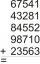Fill letters instead of digits so the indicated sum (equal letters represent equal digits). What number is hidden under the letter J? A A H A H O A H O J -------------------------- 4 3 2 1